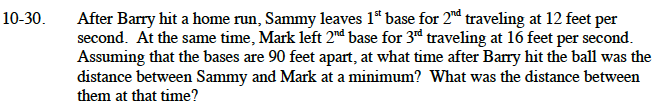### Home > CALC > Chapter 10 > Lesson 10.1.4 > Problem10-30

10-30.Sammy's distance is the horizontal leg of a right triangle. It is decreasing with time.
Write an expression for Sammy's distance (from second base).

Mark's distance is the vertictal leg of a right triangle. It is increasing with time.
Write an expression for Mark's distance (from third base).

Use the Pythagorean Theorem to write an equation for the distance between Sammy and Mark.

Differentiate your equation and set it equal to 0 to solve for the time when the distance between Sammy and Mark is a minimum.